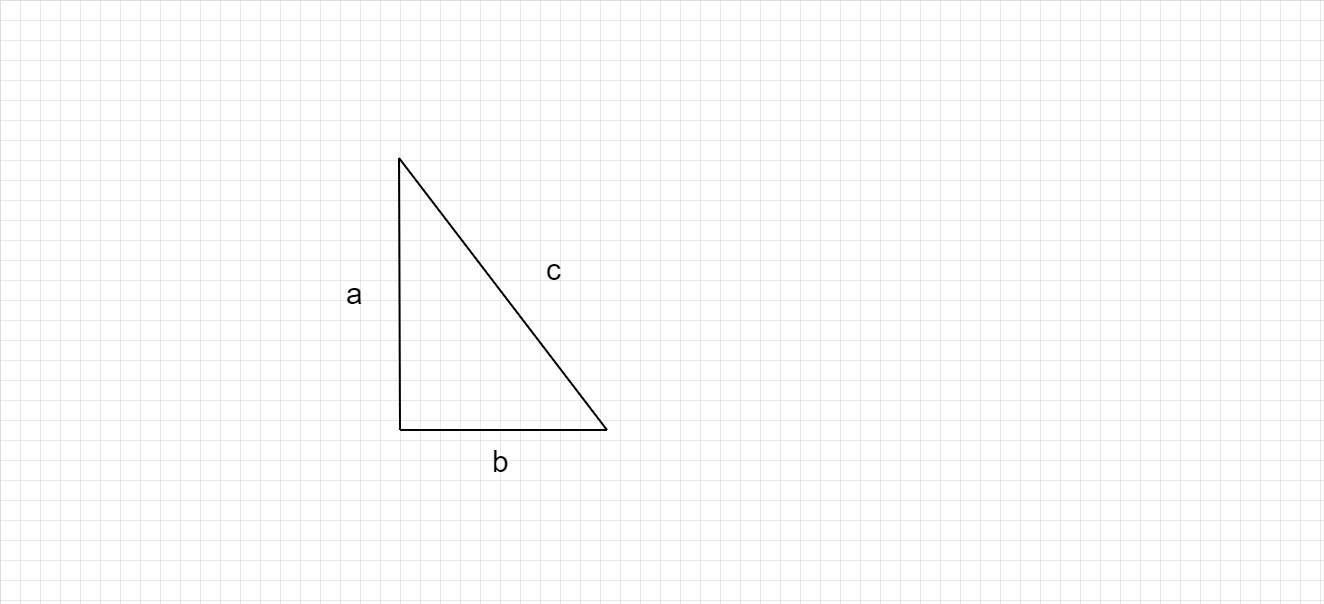# What are Pythagorean triplets ?

Pythagorean triplets :

Pythagorean triplets are set of positive integers a, b, c  that should satisfy Pythagoras theorem, $a^2 + b^2 = c^2$Consider the above right angled triangle

a, b  are length of sides

c is hypotenuse (Longest side)

Where as a, b, c are positive integers.

It will satisfy the Pythagorean formula  $a^2 + b^2 = c^2$

Lets see some examples ,

$3^2 + 4^2 = 5^2$   ;     $9 + 16 = 25$               (5 is hypotenuse 3, 4 are other sides)

$5^2 + 12^2 = 13^2$  ;  $25 + 144 = 169$       (13 is hypotenuse 5, 12 are other sides)

This is called Pythagorean Triplet.

Updated on: 10-Oct-2022

41 Views